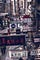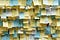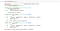# Dynamic Programming, What Is That?, Why? and How?

Dynamic programming is actually about thinking in a human way. When you have one question to answer where you can divide this to some sub-questions that you have already answers to them, you don’t have to try solve the same problems again.

Think about you need to find the market around the city. You have followed some routes, asked to locals and after some time you are at the market. Next day, you wanted to buy medicine and people said that there is a pharmacy next to the market. So at this point you have really clear understanding of pharmacy location.Photo by Arnie Chou

So how this happened? Because you have divided the problem to two sub-problems.
- Where is the market?
- Where is the pharmacy?
You have the answer of first question, you have already answered this question, have solved this question yesterday. So you don’t need to resolve this, you just need to go to the market again, and find the pharmacy next to it. And then it will become your final answer. This is all about “Dynamic Programming”.It is all about remembering! Photo by jeonsango

Here is another mathematical example. That is one of the most common examples for Dynamic Programming, so I am going to use it, Fibonacci problem. Fibonacci can be explained as,

F(0)=F(1)=1 and F(n)=F(n-1)+F(n-2), for n>1

So if you want to find F(n), first you need to solve two questions, F(n-1) and F(n-2). Some examples;

F(2) = F(1)+F(0)

F(3) = F(2) + F(1) = (F(1)+F(0)) + F(1)

F(4) = F(3) + F(2) = (F(2)+F(1)) + (F(1)+F(0))
F(4)= ((F(1)+F(0)) + F(1)) + (F(1) + F(0))

If you want to find F(3), you need to find F(2) and F(1) after that if you want to find F(4), then you need F(3) and F(2), but you already have the values of them. You don’t need to recalculate them!

Let’s see some coding after that, and it will be easier to understand differences. Let’s write basic fibonacci method. It calculates the value with recursive algorithm. As we mentioned above, this algorithm is doing all the calculations without remembering the past.

Dynamic Programming can be done in a two way, they are “Memoization” and “Tabulation”.

Memoization is a top-down approach.

When we look at this image which is a graph shown of F(5), to find F(5), respectively we need to find F(4), F(3), F(2), F(1), F(0).

Right after following this route, rest will be solved as well. So we
will not re-calculate the same values, which means we will sa ve 9 calculations in total for F(5). This is called Memoization. We are making our calculations when we need it and store them in an array to remember them. Every time we need a value, first we are checking our array, if we haven’t calculate this value, we will do our job.

On the other hand, Tabulation is a bottom-up method. We will first calculate all the values, put it to an array. And our final calculation will be done by using this array.

When you compare tabulation and memoization, both can be used in general but they have cons and pros as well. Memoization can be considered as more efficient, but when we need to find results of lot’s of operations, means lot’s of recursive operations may result failed operation.Too Many Recursive Call Example

Since tabulation is filling our array at the beginning and then calculates the result at once, tabulation can solve our problem. But remember that you have limited memory!

So it depends! You can use both methods to make your operations more efficient. Algorithms are better when they remember!

When I met with Dynamic Programming, it was a great moment for me to realize how basic it is and how efficient as well. I am suggesting that please write your own methods and give it a try to see how it makes your task easier and more efficient. It’s always better when you practice! Thank you for your time and hope to see you in my next story!

Full-time developer, and lifelong learner. Loves to learn and experience. Motto: Stay Hungry, Stay Foolish | @anilemrah_

## More from Anil Emrah

Full-time developer, and lifelong learner. Loves to learn and experience. Motto: Stay Hungry, Stay Foolish | @anilemrah_